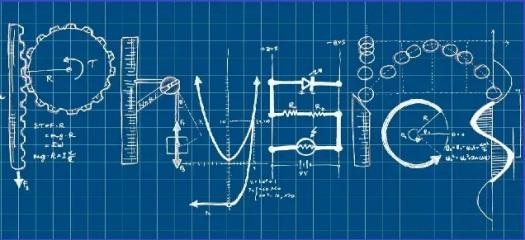# Online Preliminary Examination 2020 For B.Sc.-II Semester,Organized By Department Of Physics,Sgmm Hingna,Dist-nagpur

25 Questions | Total Attempts: 67SettingsCreate your own QuizB. Sc. Sem II Instructions: The Quiz is based on the R. T. M. Nagpur University Syllabus of B. Sc. (Physics). B. Sc. First Year (Semester II) Course – PHYSICS - Paper-I (201) (Oscillations, Kinetic theory of gases and Thermodynamics) The Quiz consist of total 25 multiple choice questions. Each question carry TWO marks All Questions are Compulsory. No negative marking Has a time limit of 120 minutes Has a pass mark of 50% You can download certificate after finishing the quiz.

• 1.
In simple harmonic (SHM), the maximum acceleration of a particle moving is
• A.

ω2/r

• B.

ω.r

• C.

ω2∙r

• D.

ω

• 2.
In a gas the transport of momentum gives rise to the phenomenon of:
• A.

Viscocity

• B.

Conduction

• C.

Diffusion

• D.

Volume

• 3.
When a rigid body is suspended vertically and it oscillates with a small amplitude under the action of the force of gravity, the body is known as
• A.

Simple pendulum

• B.

Compound pendulum

• C.

Torsional pendulum

• D.

Second’s pendulum

• 4.
Quality Factor (Q) for the Damped Oscillator is defined as:
• A.

Q = 2π (energy lost per period/ energy stored in the system)

• B.

Q = 2π (energy stored in the system x energy lost per period)

• C.

Q = 2π (energy stored in the system /energy lost per period)

• D.

Q = 2π (energy lost per period + energy stored in the system)

• 5.
Forced vibration is known as
• A.

Driven harmonic vibration

• B.

Simple harmonic vibration

• C.

Simple harmonic vibration

• D.

Free vibration

• 6.
For Boyle's law to hold good, the gas should be ___________
• A.

Real and of constant mass and temperature

• B.

Real and at constant temperature but variable mass

• C.

Perfect and of constant mass and temperature

• D.

Perfect and at constant temperature but variable mass

• 7.
The temperature of gas molecule in the container is _______ proportional to mean free path of a gas molecule.
• A.

Inversely

• B.

No effect

• C.

Directly

• D.

None of above

• 8.
A real gas most closely approaches the behavior of an ideal gas under conditions of:
• A.

Low Pressure and Temperature

• B.

Low Pressure and high Temperature

• C.

High Pressure and Temperature

• D.

High Pressure and low Temperature

• 9.
Which one do you like?
• A.

Option 1

• B.

Option 2

• C.

Option 3

• D.

Option 4

• 10.
The number of degrees of freedom of a diatomic gas molecule is:
• A.

3

• B.

4

• C.

5

• D.

6

• 11.
The term N.T.P. stands for.......
• A.

Nominal temperature and pressure

• B.

Normal thermodynamic pressure

• C.

Normal temperature and pressure

• D.

Natural temperature and pressure

• 12.
The constant used in pressure correction by Vander Waal is
• A.

A

• B.

B

• C.

C

• D.

D

• 13.
First law of thermodynamics is ________
• A.

Internal Energy= (Heat added) x (work done)

• B.

Internal Energy= (Heat added) / (work done)

• C.

Internal Energy= (Heat added) – (work done)

• D.

Internal Energy=(Heat added) + (work done)

• 14.
"If two system are both in equilibrium with a third system, they are in thermal equilibrium with each other" This is the statement of
• A.

Third law of thermodynamics

• B.

Zeroth law of thermodynamics

• C.

First law of thermodynamics

• D.

Second law of thermodynamics

• 15.
Total amount of energy in the universe is
• A.

Increasing

• B.

Decreasing

• C.

Constant

• D.

None of the above

• 16.
A reversible cycle has following processes.
• A.

• B.

4 isothermal processes

• C.

2 isothermal and 2 adiabatic processes

• D.

None of the mentioned

• 17.
An increase in enthalpy results increase in
• A.

Mass

• B.

Volume

• C.

Internal energy

• D.

Pressure

• 18.
The closed system can be represented by equation
• A.

DQ = dU x pdV

• B.

DQ = dU - pdV

• C.

DQ = dU + pdV

• D.

None of the above

• 19.
The average kinetic energy is given by_________
• A.

0.5 KT

• B.

1.5 KT

• C.

2.5 KT

• D.

3.5 KT

• 20.
When Helium expands at room temperature
• A.

Cooling is produced

• B.

Heating is produced

• C.

Neither heating nor cooling is produced

• D.

None

• 21.
"It is impossible to reduce any system to the absolute zero of temperature in a finite number of operations." This is the statement of
• A.

First law of thermodynamics

• B.

Second law of thermodynamics

• C.

Third law of thermodynamics

• D.

None of the above

• 22.
Example of reversed heat engine is
• A.

Heat pump

• B.

Refrigerator

• C.

Heat pump and Refrigerator

• D.

None of the above

• 23.
In a refrigeration system, the expansion device is connected between the
• A.

Compressor and condenser

• B.

Evaporator and compressor

• C.

• D.

• 24.
Which of the following gas will satisfy Boyle's law over a wider range of temperatures and pressures?
• A.

Hydrogen

• B.

Oxygen

• C.

Helium

• D.

Carbon monoxide

• 25.
Reynolds law hold good at constant
• A.

Pressure

• B.

Temparature

• C.

Volume

• D.

None of the above

Related TopicsBack to top
×

Wait!
Here's an interesting quiz for you.